Technical Information Site of Power Supply Design

• Basic Knowledge
• AC/DC
• Design of a Secondary-Side Synchronous Rectifying Circuit to Improve the Efficiency of an AC/DC Converter
• Shunt Regulator Circuit Section: Selection of Peripheral Circuit Components

2020.03.25 AC/DC

# Shunt Regulator Circuit Section: Selection of Peripheral Circuit Components

Design of a Secondary-Side Synchronous Rectifying Circuit to Improve the Efficiency of an AC/DC Converter

Up until the previous article, peripheral components of a synchronous rectification circuit section of the BM1R00147F power supply IC to be used were explained. In this article, we explain peripheral components of a shunt regulator circuit section.

Our discussion had focused on synchronous rectification circuits for some time, but please refer to this page to review the BM1R00147F constituent blocks.

Peripheral Components of Shunt Regulator Circuit Section

The diagram on the right shows the circuit diagram of the internal shut regulator part of the BM1R00147F and the necessary peripheral components.

First, the resistances RFB1 and RFB2 that set the output voltage VOUT are calculated. Because the internal shunt regulator uses CMOS technology, there is no need to secure an input (SH_IN) bias current for operation of the internal op-amp. Hence high resistance values can be used for RFB1 and RFB2, and by setting the current IFB that flows through the resistors RFB1 and RFB2 to the lowest limit, power consumption during standby can be reduced.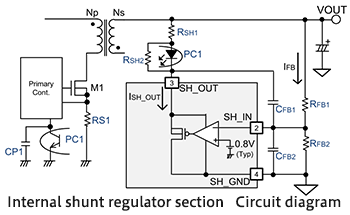If the impedance is set too high, there is the possibility of excessive sensitivity to noise and other unstable behavior; in consideration of operation stability, RFB1 and RFB2 are selected such that IFB=10 μA or so. Given the reference voltage VREF=0.8 V (Typ) for the internal shunt regulator, VOUT is determined by the following equation.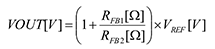In this design case study, VOUT is 5 V, and so RFB1 and RFB2 can be found using the following equation.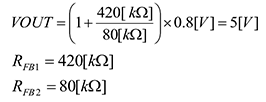We see that because IFB=10µA for VOUT=5 V, the sum of RFB1 and RFB2 is set to 500 kΩ, and the ratio of RFB1 and RFB2 should be determined such that the voltage at the branch point (SH_IN) is 0.8 V.

Next, CFB1 and CFB2 are determined. CFB1 is a capacitor for phase compensation; a value of about 1000 pF is selected. CFB2 is a capacitor for noise elimination at the SH_IN pin; typical values are in the range of about 100 to 470 pF. In this design case study, 220 pF was used.

The output load transient response can be adjusted using the resistor RSH1 to impart a bias to the photocoupler PC1. By using a small value for RSH1, the output load transient response is made fast, and output voltage load fluctuations can be suppressed. However, there is a tradeoff between the load transient response speed and stability, and so thorough verification is necessary. In this design case study, a value of 510 Ω is used.

The resistance RSH2 sets the circuit current of the internal shunt regulator. When SH_IN=Low, the maximum value ISH_OUT_max of the SH_OUT pin current is 75 μA. Hence the relationship between the Vf minimum value Vf_min of the photocoupler PC1 and the resistance RSH2 must satisfy the following inequality.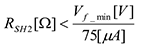When Vf_min of the photocoupler PC1 is set to 1.1 V, RSH2 is calculated using the following formula to be 14.7 kΩ or less.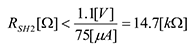In this design case study, a value of 12 kΩ is chosen, including a margin.

This concludes selection of the peripheral components of the shunt regulator circuit section.

#### Key Points:

・The output voltage is set by setting the peripheral components of the BM1R00147F shunt regulator circuit section.